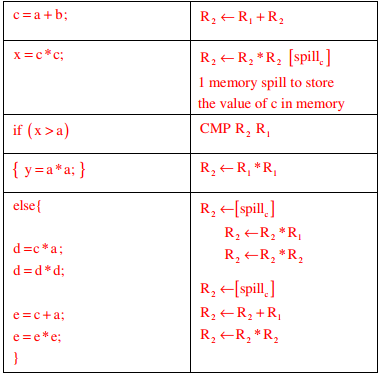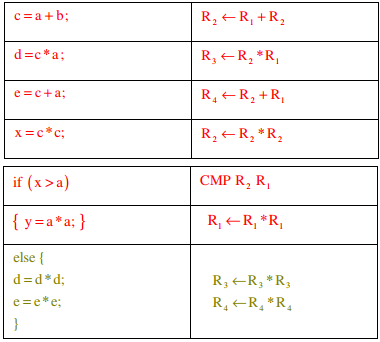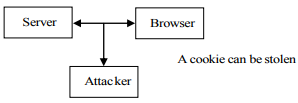# Operating System - Online Test

Q1. A shared variable x, initialized to zero, is operated on by four concurrent processes W, X, Y, Z as follows. Each of the processes W and X reads x from memory, increments by one, stores it to memory, and then terminates. Each of the processes Y and Z reads x from memory, decrements by two, stores it to memory, and then terminates. Each process before reading x invokes the P operation (i.e., wait) on a counting semaphore S and invokes the V operation (i.e., signal) on the semaphore S after storing x to memory. Semaphore S is initialized to two. What is the maximum possible value of x after all processes complete execution?
Explaination / Solution:
No Explaination.

Q2. The following code segment is executed on a processor which allows only register operands in its instructions. Each instruction can have almost two source operands and one destination operand.
Assume that all variables are dead after this code segment
c = a + b;
d = c * a;
e = c + a;
x = c *c;
if (x > a) {
y = a * a;
}
else {
d = d * d;
e = e * e;
}
Suppose the instruction set architecture of the processor has only two registers. The only allowed compiler optimization is code motion, which moves statements from one place to another while preserving correctness. What is the minimum number of spills to memory in the compiled code?
Explaination / Solution:

After applying the code motion optimization the statement d=c*a; and e=c+a; can be moved down to else block as d and e are not used anywhere before that and also value of a and c is not changing.In the above code total number of spills to memory is 1

Q3. The following code segment is executed on a processor which allows only register operands in its instructions. Each instruction can have almost two source operands and one destination operand.
Assume that all variables are dead after this code segment
c = a + b;
d = c * a;
e = c + a;
x = c *c;
if (x > a) {
y = a * a;
}
else {
d = d * d;
e = e * e;
}
What is the minimum number of registers needed in the instruction set architecture of the processor to compile this code segment without any spill to memory? Do not apply any optimization other than optimizing register allocation
Explaination / Solution:In the above code minimum number of registers needed are = 4

Q4.  Which of the following statements are CORRECT?
1) Static allocation of all data areas by a compiler makes it impossible to implement recursion.
2) Automatic garbage collection is essential to implement recursion.
3) Dynamic allocation of activation records is essential to implement recursion.
4) Both heap and stack are essential to implement recursion.
Explaination / Solution:

To implement recursion, activation record should be implemented by providing dynamic memory allocation. This dynamic allocation is done from runtime stack. Heap is essential to allocate memory for data structures at run-time, not for recursion. So statement 1 and 3 are correction.

Q5. In the context of modular software design, which one of the following combinations is desirable?
Explaination / Solution:

Cohesion is a measure of internal strength within a module, whereas coupling is a measure of inter dependency among the modules. So in the context of modular software design there should be high cohesion and low coupling.

Q6. Identify the correct order in which a server process must invoke the function calls accept, bind, listen, and recv according to UNIX socket APL
Explaination / Solution:

The correct order in which a server process must invoke the function calls is bind, listen, accept and recv. First three are used in connection establishment phase and recv is used in data transfer phase.

Q7. Which one of the following statements is NOT correct about HTTP cookies?
Explaination / Solution:

(A) is correct
(B) Option B is false
(C) Option C is correct
(D) Option D is correctQ8. Consider six memory partitions of sizes 200 KB, 400 KB, 600 KB, 500 KB, 300 KB and 250 KB, where KB refers to kilobyte. These partitions need to be allotted to four processes of sizes 357 KB, 210KB, 468 KB and 491 KB in that order. If the best fit algorithm is used, which partitions are NOT allotted to any process?
Explaination / Solution:Q9. Consider an arbitrary set of CPU-bound processes with unequal CPU burst lengths submitted at the same time to a computer system. Which one of the following process scheduling algorithms would minimize the average waiting time in the ready queue?
Explaination / Solution:

SRTF is pre emptive SJF which produces less average waiting time.

Q10. A multithreaded program P executes with x number of threads and uses y number of locks for ensuring mutual exclusion while operating on shared memory locations. All locks in the program are non-reentrant, i.e., if a thread holds a lock l, then it cannot re-acquire lock l without releasing it. If a thread is unable to acquire a lock, it blocks until the lock becomes available. The minimum value of x and the minimum value of y together for which execution of P can result in a deadlock are:
Explaination / Solution:

As per given question, there 'x' number of threads and 'y' number of locks for ensuring mutual exclusion while operating on shared memory locations
Option (A): x=1;y=2
Means that 1 thread and 2 locks clearly showing that no deadlock situation
Option (B): x=2;y=1
After usage of lock by 1 thread, it can release that lock and then 2nd thread can be used that lock. So no deadlock
Option(C):x=2;y=2
Both threads can hold 1 lock and can wait for release of another lock
Option(D) x=1; y=1